Nuclear equations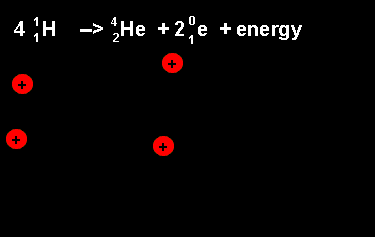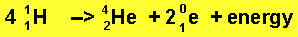The equation above outlines the formation of a helium nucleus. The number of nucleons remains the same on both sides. When four protons fuse a helium nucleus is formed, energy given off and 2 positrons ejected. The ejection of two positrons turns two protons into neutrons. The animation on the left shows this reaction. The animation on the right shows the nuclear fission reaction of lithium. The lithium nucleus absorbs a neutron and splits into a hydrogen and helium nucleus(alpha particle). Once again note that the number of nucleons is the same on both sides of the equation.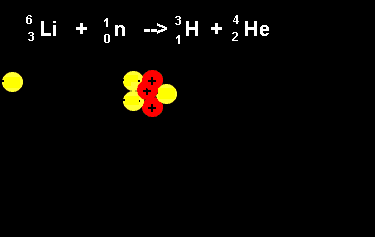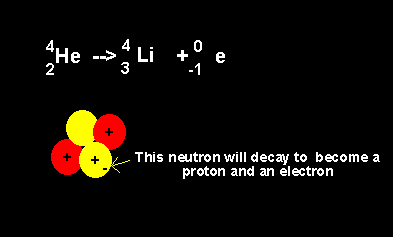Helium decays to form a lithium nucleus with the release of an electron. Notice the number of nucleons is the same on both sides of the equation. A neutron decays with the release of an electron to form a proton. The atomic number increases from 2 to 3 but the atomic mass number remains the same at 4 nucleons. In summary: -a positron is emitted when a proton decays to a neutron -an electron is emitted when a neutron decays to a proton. Click for more information. Identify "X" in the following nuclear reactions.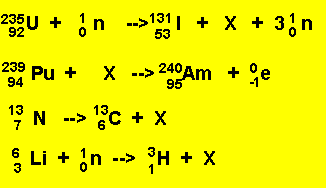Solution Continue with more exercises.

Home